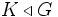# Upward-closed normal subgroup

This article defines a subgroup property: a property that can be evaluated to true/false given a group and a subgroup thereof, invariant under subgroup equivalence. View a complete list of subgroup properties[SHOW MORE]

This property of a normal subgroup is completely characterized by the abstract isomorphism class of the quotient group

BEWARE! This term is nonstandard and is being used locally within the wiki. [SHOW MORE]

## Definition

### Symbol-free definition

A subgroup of a group is termed upward-closed normal if it satisfies the following equivalent conditions:

• Every subgroup intermediate between the given subgroup and the whole group, is normal in the whole group.
• It is normal and the quotient group is a Dedekind group, viz all subgroups of the quotient group are normal in it.

### Definition with symbols

A subgroup$H$ of a group$G$ is termed upward-closed normal if it satisfies the following equivalent conditions:

• For any subgroup$K$ such that$H \le K \le G$,$K \triangleleft G$.
•$H \triangleleft G$, and the quotient group$G/H$ is a Dedekind group, viz., all its subgroups are normal.

### In terms of the upward closure operator

The property of being an upward-closed normal subgroup is obtained by applying the upward closure operator to the subgroup property of being normal.

## Metaproperties

### Transitivity

NO: This subgroup property is not transitive: a subgroup with this property in a subgroup with this property, need not have the property in the whole group
ABOUT THIS PROPERTY: View variations of this property that are transitive|View variations of this property that are not transitive
ABOUT TRANSITIVITY: View a complete list of subgroup properties that are not transitive|View facts related to transitivity of subgroup properties | View a survey article on disproving transitivity

Clearly, an upward-closed normal subgroup of an upward-closed normal subgroup need not be normal. In fact, in any solvable non-Abelian group, the second derived subgroup is an upward-closed normal subgroup of an upward-closed normal subgroup, yet it is usually not itself upward-closed normal.

### Intermediate subgroup condition

YES: This subgroup property satisfies the intermediate subgroup condition: if a subgroup has the property in the whole group, it has the property in every intermediate subgroup.
ABOUT THIS PROPERTY: View variations of this property satisfying intermediate subgroup condition | View variations of this property not satisfying intermediate subgroup condition
ABOUT INTERMEDIATE SUBROUP CONDITION:View all properties satisfying intermediate subgroup condition | View facts about intermediate subgroup condition

The property of being upward-closed normal satisfies the intermediate subgroup condition, viz if$H \le K \le G$ and$H$ is upward-closed normal in$G$,$H$ is also upward-closed normal in$K$. This follows essentially from the fact that normality satisfies the intermediate subgroup condition.

### Trimness

The property of being upward-closed normal is not trivially true. However, it is an identity-true subgroup property, viz every group is upward-closed normal as a subgroup of itself.